2021 NFL Defensive Rookie of the Year Odds
+600
6 to 1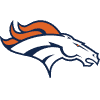Patrick Surtain
8.4% implied probability

+650
6.5 to 1Micah Parsons
7.9% implied probability

+800
8 to 1Jamin Davis
6.5% implied probability

+1000
10 to 1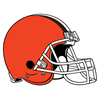Jeremiah Owusu-Koramoah
5.4% implied probability

+1200
12 to 1Zaven Collins
4.5% implied probability

+1200
12 to 1Jaycee Horn
4.5% implied probability

+1200
12 to 1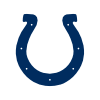Kwity Paye
4.5% implied probability

+1600
16 to 1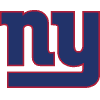Azeez Ojulari
3.5% implied probability

+2000
20 to 1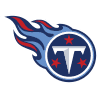Caleb Farley
2.8% implied probability

+2200
22 to 1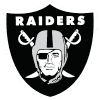Trevon Moehrig
2.6% implied probability

+2200
22 to 1Joe Tryon
2.6% implied probability

+2500
25 to 1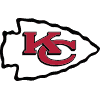Nick Bolton
2.3% implied probability

+2800
28 to 1Greg Newsome
2.0% implied probability

+3000
30 to 1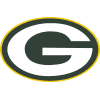Eric Stokes
1.9% implied probability

+3500
35 to 1Jabril Cox
1.6% implied probability

+3500
35 to 1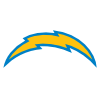Asante Samuel
1.6% implied probability

+3500
35 to 1Payton Turner
1.6% implied probability

No changes have been recorded yet.
+4000
40 to 1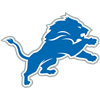Derrick Barnes
1.4% implied probability

No changes have been recorded yet.
+4000
40 to 1Ronnie Perkins
1.4% implied probability

+4000
40 to 1Baron Browning
1.4% implied probability

+4000
40 to 1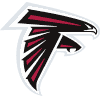Richie Grant
1.4% implied probability

+4000
40 to 1Elijah Molden
1.4% implied probability

No changes have been recorded yet.
+4000
40 to 1Christian Barmore
1.4% implied probability

+4000
40 to 1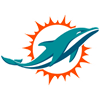Jevon Holland
1.4% implied probability

+4000
40 to 1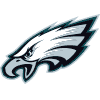Milton Williams
1.4% implied probability

No changes have been recorded yet.
+5000
50 to 1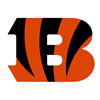Joseph Ossai
1.2% implied probability

+5000
50 to 1Justin Hilliard
1.2% implied probability

No changes have been recorded yet.
+5000
50 to 1Rashad Weaver
1.2% implied probability

No changes have been recorded yet.
+5000
50 to 1Pete Werner
1.2% implied probability

+5000
50 to 1Levi Onwuzurike
1.2% implied probability

No changes have been recorded yet.
+5000
50 to 1Carlos Basham
1.2% implied probability

No changes have been recorded yet.
+6500
65 to 1Tommy Togiai
0.9% implied probability

No changes have been recorded yet.
+6500
65 to 1Ar'Darius Washington
0.9% implied probability

No changes have been recorded yet.
+6500
65 to 1Jamar Johnson
0.9% implied probability

No changes have been recorded yet.
+6500
65 to 1Daviyon Nixon
0.9% implied probability

No changes have been recorded yet.
+6500
65 to 1Tay Gowan
0.9% implied probability

No changes have been recorded yet.
+6500
65 to 1Osa Odighizuwa
0.9% implied probability

No changes have been recorded yet.
+6500
65 to 1Andre Cisco
0.9% implied probability

No changes have been recorded yet.
+6500
65 to 1Chazz Surratt
0.9% implied probability

+6500
65 to 1Aaron Robinson
0.9% implied probability

+6500
65 to 1Kelvin Joseph
0.9% implied probability

+6500
65 to 1Ifeatu Melifonwu
0.9% implied probability

No changes have been recorded yet.
+6500
65 to 1Bobby Brown
0.9% implied probability

No changes have been recorded yet.
+8000
80 to 1Marvin Wilson
0.7% implied probability

No changes have been recorded yet.
+10000
100 to 1Nate Hobbs
0.6% implied probability

No changes have been recorded yet.
+10000
100 to 1Thomas Graham
0.6% implied probability

No changes have been recorded yet.
+10000
100 to 1Divine Deablo
0.6% implied probability

No changes have been recorded yet.
+10000
100 to 1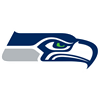Tre Brown
0.6% implied probability

No changes have been recorded yet.
+10000
100 to 1Benjamin St-Juste
0.6% implied probability

No changes have been recorded yet.
+10000
100 to 1Tyler Shelvin
0.6% implied probability

No changes have been recorded yet.
+10000
100 to 1Tyson Campbell
0.6% implied probability

No changes have been recorded yet.
+10000
100 to 1Dayo Odeyingbo
0.6% implied probability

No changes have been recorded yet.
+10000
100 to 1Cameron Sample
0.6% implied probability

No changes have been recorded yet.
+10000
100 to 1Hamsah Nasirildeen
0.6% implied probability

No changes have been recorded yet.